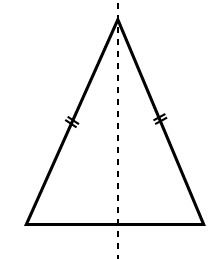# Selina Solutions Concise Maths Class 7 Chapter 17: Symmetry (Including Reflection and Rotation) Exercise 17C

Selina Solutions Concise Maths Class 7 Chapter 17 Symmetry (Including Reflection and Rotation) Exercise 17C has answers designed by teachers having experience in the education industry. The PDF of solutions has stepwise explanations to boost confidence among students to perform well in the annual exam. Pictorial representation of solutions mainly improves conceptual knowledge among students. The main objective of providing the exercise wise solutions is to help students understand the concepts, irrespective of their intelligence quotient. Selina Solutions Concise Maths Class 7 Chapter 17 Symmetry (Including Reflection and Rotation) Exercise 17C PDF, links which are given here for free download.

## Selina Solutions Concise Maths Class 7 Chapter 17: Symmetry (Including Reflection and Rotation) Exercise 17C Download PDF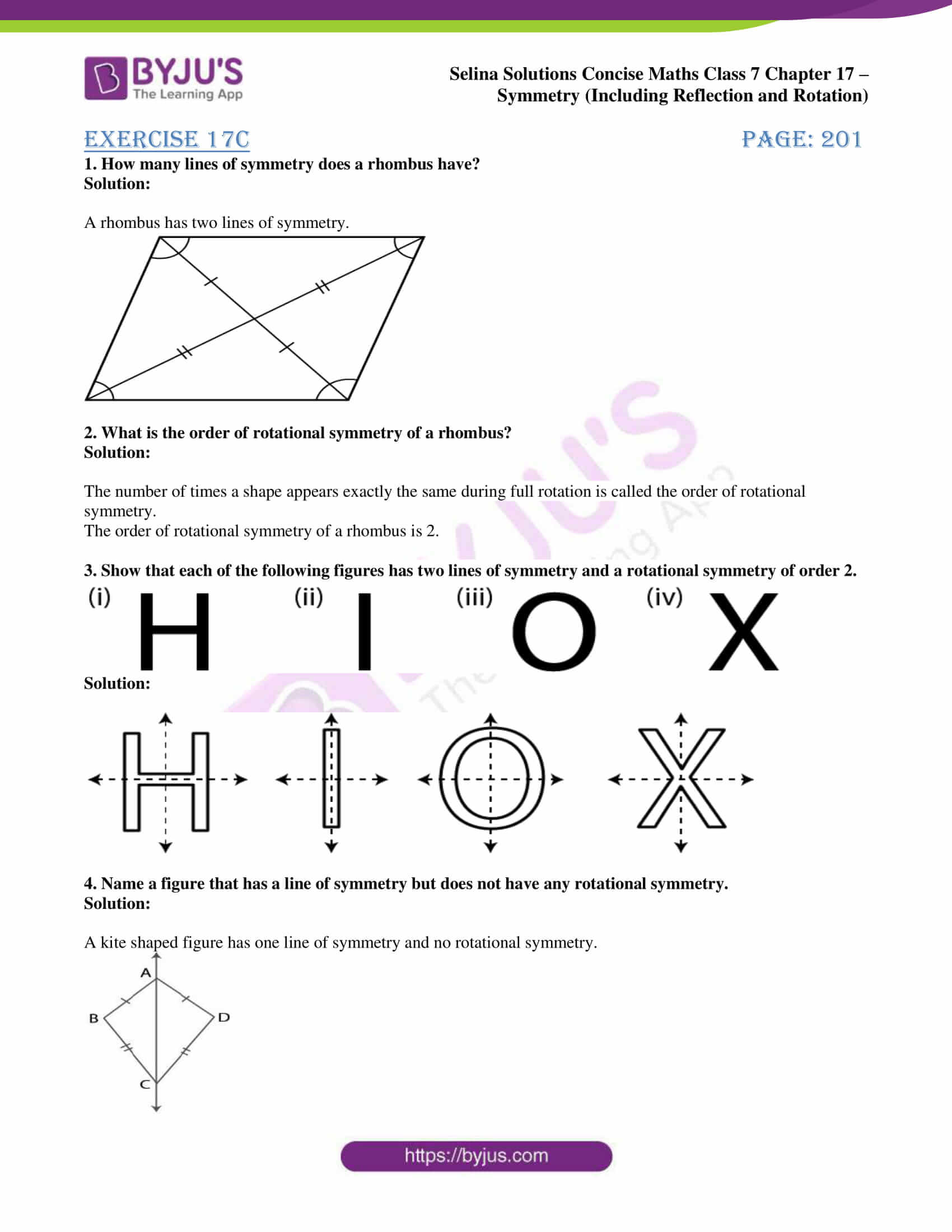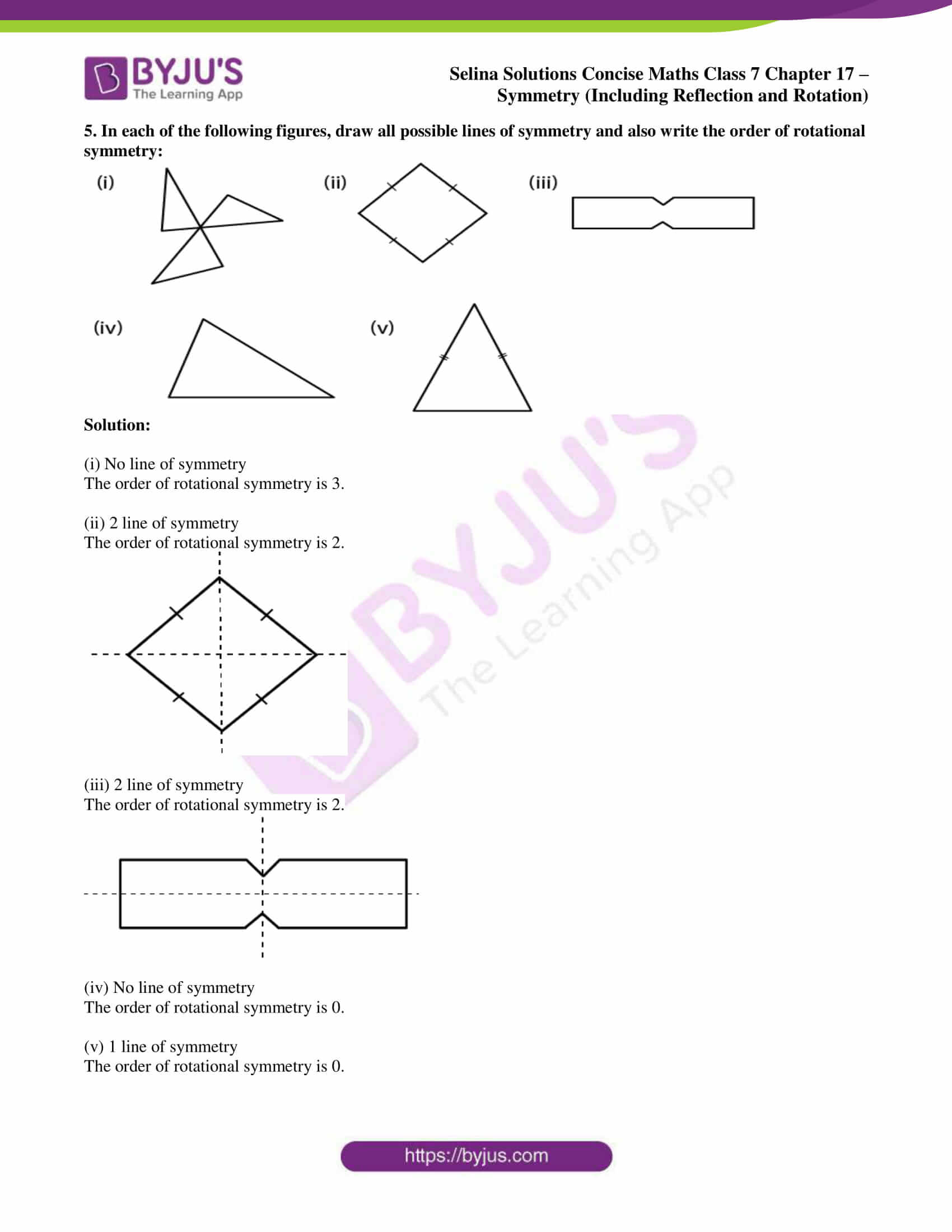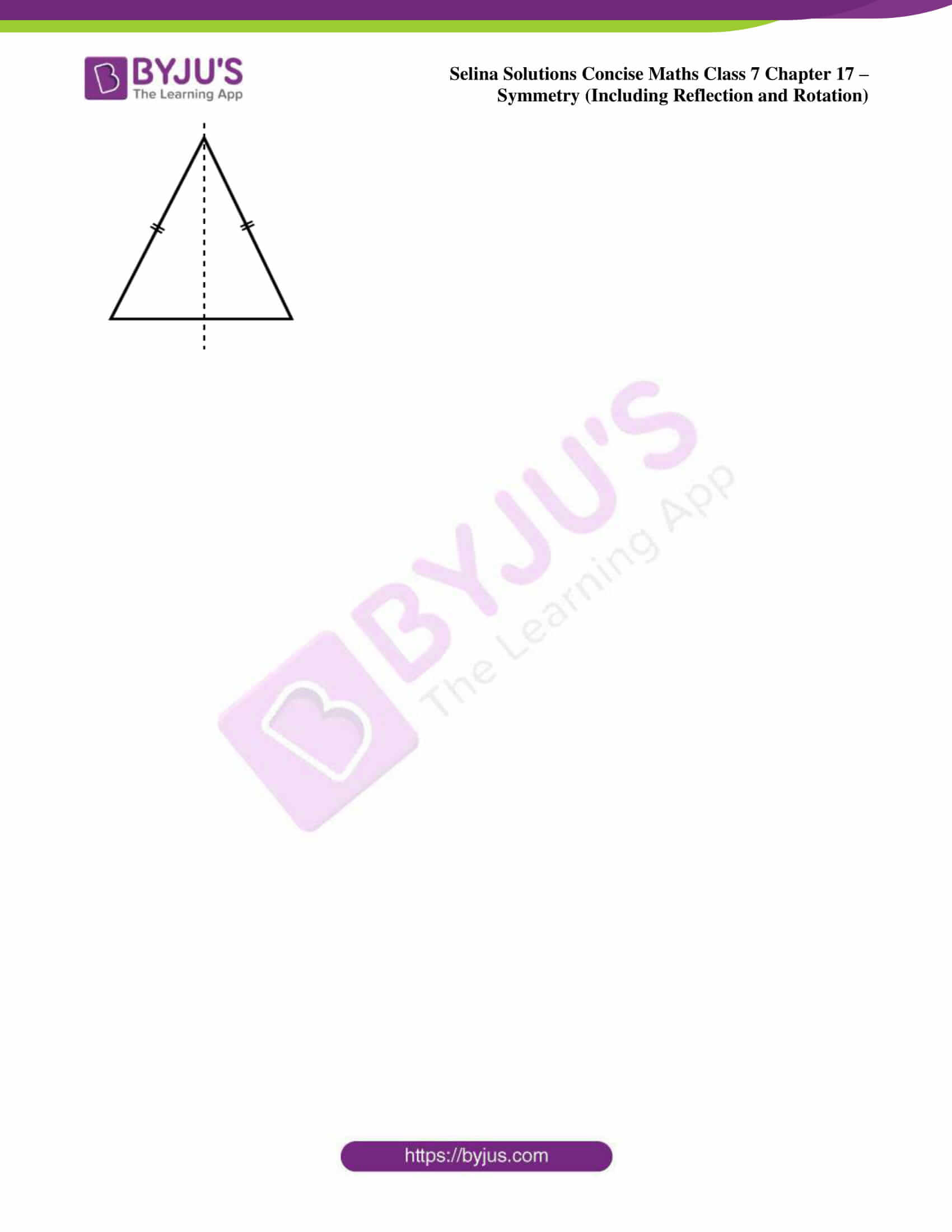### Access other exercises of Selina Solutions Concise Maths Class 7 Chapter 17: Symmetry (Including Reflection and Rotation)

Exercise 17A Solutions

Exercise 17B Solutions

### Access Selina Solutions Concise Maths Class 7 Chapter 17: Symmetry (Including Reflection and Rotation) Exercise 17C

#### Exercise 17C page: 201

1. How many lines of symmetry does a rhombus have?

Solution:

A rhombus has two lines of symmetry.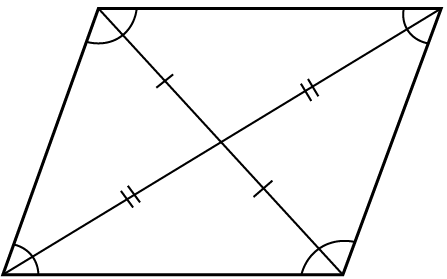2. What is the order of rotational symmetry of a rhombus?

Solution:

The number of times a shape appears exactly the same during full rotation is called the order of rotational symmetry.

The order of rotational symmetry of a rhombus is 2.

3. Show that each of the following figures has two lines of symmetry and a rotational symmetry of order 2.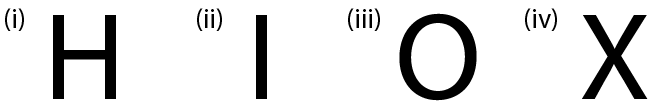Solution: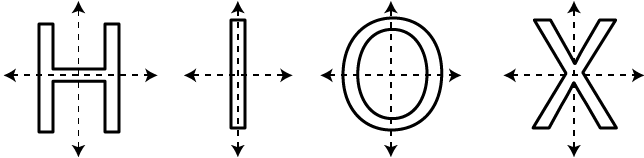4. Name a figure that has a line of symmetry but does not have any rotational symmetry.

Solution:

A kite shaped figure has one line of symmetry and no rotational symmetry.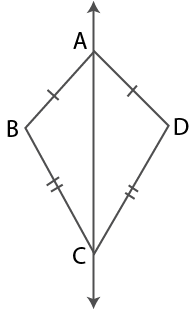5. In each of the following figures, draw all possible lines of symmetry and also write the order of rotational symmetry: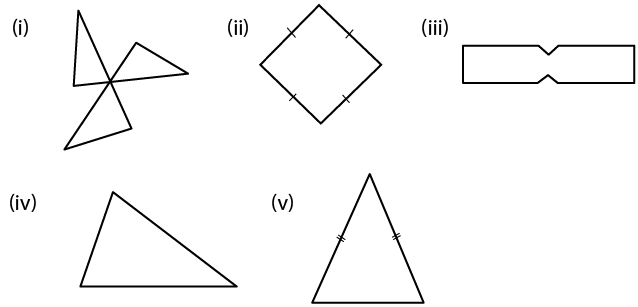Solution:

(i) No line of symmetry

The order of rotational symmetry is 3.

(ii) 2 line of symmetry

The order of rotational symmetry is 2.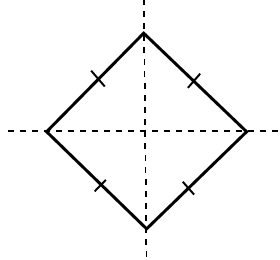(iii) 2 line of symmetry

The order of rotational symmetry is 2.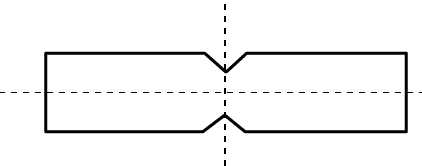(iv) No line of symmetry

The order of rotational symmetry is 0.

(v) 1 line of symmetry

The order of rotational symmetry is 0.